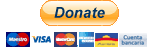# skforecast¶

Time series forecasting with scikit-learn regressors.

Skforecast is a python library that eases using scikit-learn regressors as multi-step forecasters. It also works with any regressor compatible with the scikit-learn API (pipelines, CatBoost, LightGBM, XGBoost, Ranger...).

Info

Version 0.4 has undergone huge code refactoring. Major changes are related to input-output formats (only pandas series and dataframes are allowed although internally numpy arrays are used for performance) and model validation methods (unified into backtesting with and without refit). All notable changes are listed in Releases.

## Installation¶

 `1` ``````pip install skforecast ``````

Specific version:

 `1` ``````pip install skforecast==0.4.2 ``````

Latest (unstable):

 `1` ``````pip install git+https://github.com/JoaquinAmatRodrigo/skforecast#master ``````

## Dependencies¶

 ```1 2 3 4 5``` ``````numpy>=1.20, <=1.22 pandas>=1.2, <=1.4 tqdm>=4.57.0, <=4.62 scikit-learn>=1.0 statsmodels>=0.12, <=0.13 ``````

## Features¶

• Create recursive autoregressive forecasters from any regressor that follows the scikit-learn API
• Create multi-output autoregressive forecasters from any regressor that follows the scikit-learn API
• Include exogenous variables as predictors
• Include custom predictors (rolling mean, rolling variance ...)
• Multiple backtesting methods for model validation
• Grid search to find optimal lags (predictors) and best hyperparameters
• Include custom metrics for model validation and grid search
• Prediction interval estimated by bootstrapping
• Get predictor importance
• Forecaster in production

## Donating¶

If you found skforecast useful, you can support us with a donation. Your contribution will help to continue developing and improving this project. Many thanks!